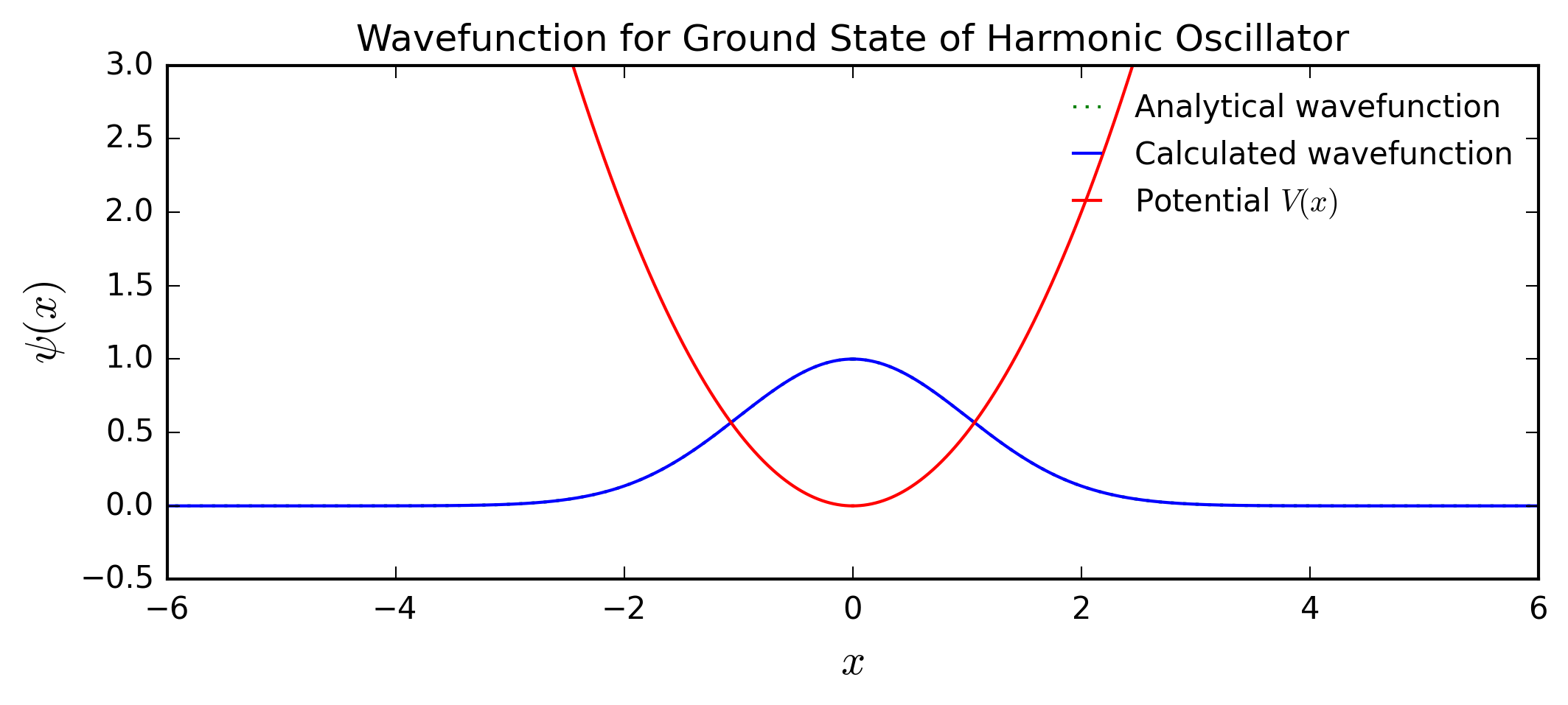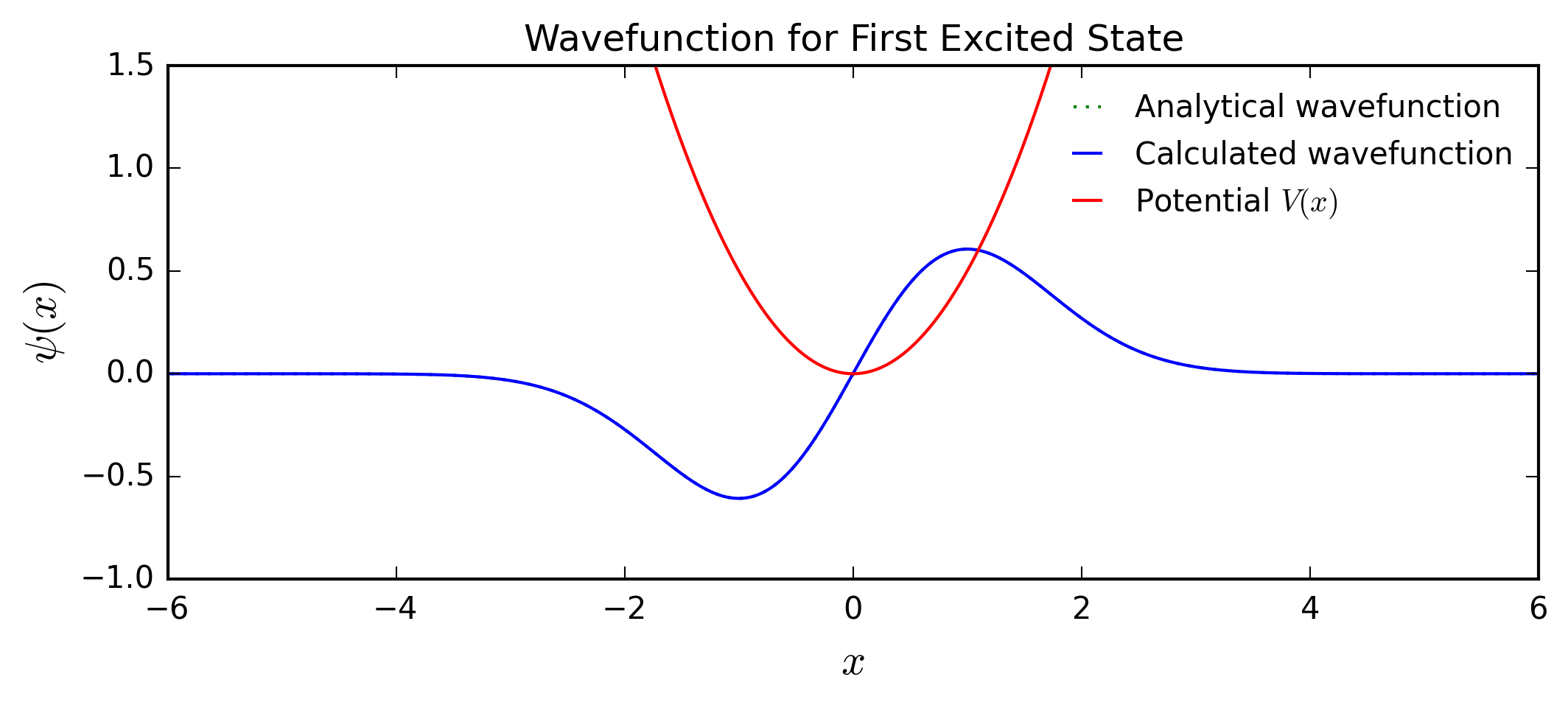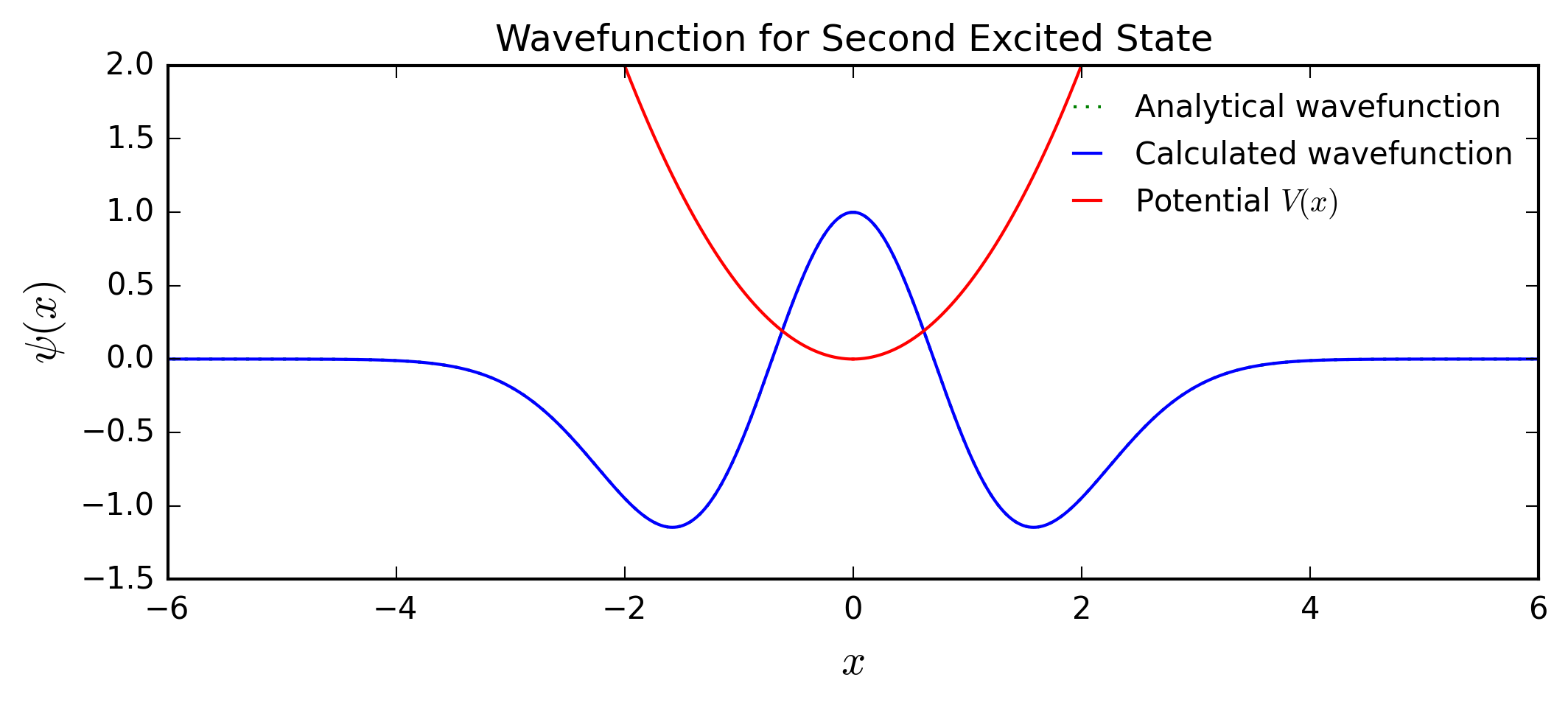# Numerical Determination of Eigenenergies for Harmonic Oscillator¶

### Examples – Quantum Mechanics¶

Last edited: April 20th 2016

The objective of this module is to use the method of forward shooting to determine numerically the eigenenergies of the quantum harmonic oscillator in one dimension. In other words, we are looking for eigenvalues of the time-independent Schrödinger equation

$$\qquad\left[-\frac{\hbar^2}{2m}\frac{\rm{d}^2}{\rm{d} x^2}+V(x)\right] \psi(x) = E \psi(x)$$

with

$$\qquad V=\frac{1}{2}m \omega^2 x^2.$$

We will focus mainly on the three lowest eigenenergies. This will demonstrate how "an eigenvalue has just the right value" to solve the time-independent Schrödinger equation. Moreover, this example shows that the forward-shooting method also works for potentials $V(x)$ for which we do not have closed-form solutions.

First, we write the Schrödinger equation in the following form

$$\qquad\psi''(x) = -\frac{2m}{\hbar^2}\left[E-\frac{1}{2}m \omega^2 x^2 \right]\psi(x),$$

and for simplicity, we set the values $m=\omega=\hbar=1$ which yields the equation

$$\qquad\psi''(x) = \left[ x^2-2E\right] \psi(x).$$

### Forward Shooting¶

We will now compute the eigenenergies of this problem by using the forward shooting method, knowing that the wave function must tend to zero as $x\to\infty$. First, we divide the interval we are interested in, $[a,b]$, into $n$ equal intervals with length

$$\qquad \Delta x = \frac{b-a}{n}$$

which defines the coordinates $x_i = a + i\Delta x$. Hence, it is natural to write the function values of $\psi(x)$, $\psi(x)'$ and $\psi(x)''$ at a point $x = x_i$ as $\psi_i$, $\psi'_i$ and $\psi''_i$, respectively.

Next, we approximate $\psi''(x)$ at a point $x_i$ by using the second-order central difference method which yields

$$\qquad \psi_i'' = \frac{\psi_{i+1}+\psi_{i-1} - 2\psi_{i}}{(\Delta x)^2}.$$

Using the simplified Schrödinger equation,

$$\qquad \psi_i'' = \left[x_i^2-2E\right]\psi_i,$$

we obtain

$$\qquad \frac{\psi_{i+1}+\psi_{i-1} - 2\psi_{i}}{(\Delta x)^2} = \left[x_i^2-2E\right]\psi_i.$$

By rearranging the previous equation, we find an equation for the function value $\psi_{i+1}$ based on the values of the previous two points

$$\qquad \psi_{i+1} = -\psi_{i-1} + \left[2 + (\Delta x)^2 \left(x_i^2-2E\right)\right] \psi_i.$$

By using this formula, we can calculate iteratively all function values as long as we have two initial values to start with. In this problem, we consider a potential which is symmetric about $x=0$. Therefore, the initial values $\psi_0$ and $\psi_0'$ will be given at $x=0$ and we approximate the next function value $\psi_1$ simply by

$$\qquad \psi_1 = \psi_0 + \Delta x \cdot \psi_0'.$$

This then allows us to calculate $\psi_i$ for higher values of $i$ using the formula above.

We know that for a symmetric potential, the ground state, second excited state, fourth excited state etc. are symmetric, while the first excited state, third excited state etc. are anti-symmetric. Since the harmonic oscillator potential is symmetric about $x=0$, it allows us to use the method in only one direction, focusing on $x>0$ and starting with the initial values at $x = 0$, namely $\psi_0$ and $\psi_0'$.

### Ground State¶

Since the ground state should be symmetric about $x=0$, we have to have $\psi_0' = 0$. We also have to choose a value for $\psi_0$, e.g. $\psi_0=1$. The particular choice of the value of $\psi_0$ will not affect the energy eigenvalue because its actual value will be determined by normalization of the wave function. Using these two initial values, we can now compute the ground state energy as follows. First, we choose an upper and a lower bound for the estimated ground state energy. This is where uncertainty arises since we do not know a priori where the eigenenergies lie. Subsequently, we keep shooting forward to $x_i=b$, starting at $x_0=0$, with an estimated eigenenergy E0 that is determined iteratively by the bisection method: if the value of the wave function grows to infinity as $x\to\infty$, we need to raise the value of E0; if the value of the wave function goes to negative infinity, we need to lower E0. We truncate the bisection method when a desired accuracy acc is achieved and plot the analytical solution versus the numerical solution of the wave function. The agreement is quite impressive.

In :
%matplotlib inline
import numpy as np
import matplotlib.pyplot as plt

# Set common figure parameters:
newparams = {'axes.labelsize': 14, 'axes.linewidth': 1, 'savefig.dpi': 300,
'lines.linewidth': 1.0, 'figure.figsize': (8, 3),
'figure.subplot.wspace': 0.4,
'ytick.labelsize': 10, 'xtick.labelsize': 10,
'legend.fontsize': 10, 'legend.frameon': False,
'legend.handlelength': 1.5}
plt.rcParams.update(newparams)

In :
n = 1000.0        # number of points per unit on the x-axis.
p = 10.0          # the number of points included on the x-axis
dx = 1.0/n        # step length

f0= np.zeros(p*n) # array for function values of f0
f0 = 1.0       # initial value for f0 at x = 0
df0_0 = 0.0       # initial value for df0/dx at x = 0

x = np.linspace(0,p,p*n,True)

acc = 1e-15       # Accuracy of eigenenergy
e1 = 0.0          # Lower bound, must be positive since the potential is positive for all x
e2 = 4.0          # Upper bound
E0 = e1
deltaE0 = 1
while deltaE0 > acc:
for i, x_ in enumerate(x[0:-1]):
if i == 0:
f0[i+1] = f0[i]+dx*df0_0
else:
f0[i+1] = -f0[i-1]+f0[i]*(2-dx**2*(2*E0-x_**2))
# Implementation of bisection method. If the function value is out of bounds,
# a new value for the energy is chosen. When the difference between the upper
# and lower bound for the energy is smaller than the given accuracy,
# the while loop stops, yielding a result for E1.
if f0[i] > 5:
e1 = E0
E0 = e1 + (e2-e1)/2
break
elif f0[i] < -5:
e2 = E0
E0 = e2 - (e2-e1)/2
break
deltaE0 = e2-e1
print(r'The energy E0 is: %s'% E0)

#Plot:
p1, = plt.plot(x, np.exp(-x**2/2), 'g:')       # analytical eigenfunction
p2, = plt.plot(x, f0, 'b')                     # computed eigenfunction
p3, = plt.plot(x, 0.5*x**2, 'r')               # potential
plt.plot(-x, np.exp(-x**2/2), 'g:', -x, f0, 'b', -x,0.5*x**2, 'r') # same as above for negative x-values
plt.legend([p1, p2, p3],['Analytical wavefunction', 'Calculated wavefunction', r'Potential $V(x)$'])
plt.xlabel(r'$x$')
plt.ylabel(r'$\psi(x)$')
plt.ylim([-0.5, 3])
plt.xlim([-6, 6])
plt.title('Wavefunction for Ground State of Harmonic Oscillator');

The energy E0 is: 0.500332214879553### First Excited State¶

For the first excited state, we have to choose different initial values. Since this is an anti-symmetric function, we must have $\psi_0=0$, while $\psi_0'$ can be chosen freely this time, e.g. $\psi_0'=1$. Again, this is only a matter of normalization. Since we are looking for the first excited state, we know that $E_1>E_0$, which means we can choose $E_0$ as our lower bound. Choosing an appropriate upper bound can be the tricky part since it should be lower than the eigenenergy of the second excited state. In any event, the agreement between the numerical and the analytical solution is again impressive.

In :
f1 = np.zeros(p*n)
f1 = 0.0
df1_0 = 1.0

e1 = E0
e2 = 2.0
E1 = e1
deltaE1 = 1
while deltaE1 > acc:
for i, x_ in enumerate(x[0:-1]):
if i == 0:
f1[i+1] = f1[i]+dx*df1_0
else:
f1[i+1] = -f1[i-1]+f1[i]*(2-dx**2*(2*E1-x_**2))
if f1[i] > 5:
e1 = E1
E1 = e1 + (e2-e1)/2
break
elif f1[i] < -5:
e2 = E1
E1 = e2 - (e2-e1)/2
break
deltaE1 = e2-e1

print(r'The energy E1 is: %s' % E1)

p1, = plt.plot(x, x*np.exp(-x**2/2), 'g:')
p2, = plt.plot(x, f1, 'b')
p3, = plt.plot(x, 0.5*x**2, 'r')
plt.plot(-x, -x*np.exp(-x**2/2), 'g:', -x, -f1, 'b', -x, 0.5*x**2, 'r')
plt.legend([p1, p2, p3],['Analytical wavefunction', 'Calculated wavefunction', r'Potential $V(x)$'])
plt.xlabel(r'$x$')
plt.ylabel(r'$\psi(x)$')
plt.ylim([-1,1.5])
plt.xlim([-6,6])
plt.title('Wavefunction for First Excited State');

The energy E1 is: 1.5001498587221596### Second Excited State¶

For the second excited state, we know that $E_2 > E_1$, and that the wavefunction is symmetric. Hence, we can use the same initial conditions as for the ground state. Again, choosing an appropriate upper limit is critical.

In :
f2 = np.zeros(p*n)
f2 = 1.0
df2_0 = 0.0

e1 = E1
e2 = 3.0
E2 = e1
deltaE2 = 1
while deltaE2 > acc:
for i, x_ in enumerate(x[0:-1]):
if i == 0:
f2[i+1] = f2[i]+dx*df2_0
else:
f2[i+1] = -f2[i-1]+f2[i]*(2-dx**2*(2*E2-x_**2))
if f2[i] < -5:
e1 = E2
E2 = e1 + (e2-e1)/2
break
elif f2[i] > 5:
e2 = E2
E2 = e2 - (e2-e1)/2
break
deltaE2 = e2-e1

# Notice that the two last conditions here are different from the two above!
# The reason is that the wavefunction now has roots for x ≠ 0, and approaches the x-axis from below.

print(r'The energy E2 is: %s' % E2)

p1, = plt.plot(x, -(2*x**2-1)*np.exp(-x**2/2), 'g:')
p2, = plt.plot(x, f2, 'b')
p3, = plt.plot(x, 0.5*x**2, 'r')
plt.plot(-x, -(2*x**2-1)*np.exp(-x**2/2), 'g:', -x, f2, 'b', -x,0.5*x**2, 'r')
plt.legend([p1, p2, p3],['Analytical wavefunction', 'Calculated wavefunction', r'Potential $V(x)$'])
plt.xlabel(r'$x$')
plt.ylabel(r'$\psi(x)$')
plt.ylim([-1.5,2])
plt.xlim([-6,6])
plt.title('Wavefunction for Second Excited State');

The energy E2 is: 2.5009550642267624From the analytical solution of the harmonic oscillator potential, we know that the eigenenergies are $$\qquad E_n^a = \hbar \omega (n + \frac{1}{2}).$$ With $\hbar = \omega = 1$, we find $E_n^a = (n+1/2)$, which is in excellent agreement with the results obtained above! We also see from the plots of the eigenfunctions that they are in excellent agreement with the analytical eigenfunctions. This is all the more surprising since we used a simple numerical scheme. Consequently, the reasons why the results deviate slightly, are mostly due to numerical errors.